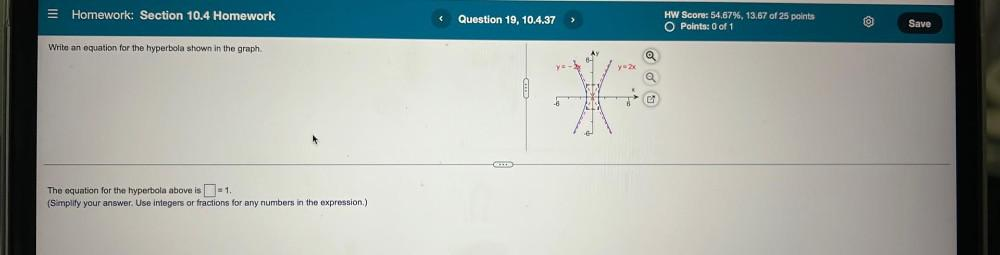Question:

# Homework: Section 10.4 Homework < Question 19, 10.4.37 > HW Score: 54.67%, 13.67 of 25 points O Points: 0 of 1 Save Write an equHomework: Section 10.4 Homework < Question 19, 10.4.37 > HW Score: 54.67%, 13.67 of 25 points O Points: 0 of 1 Save Write an equation for the hyperbola shown in the graph. y = 2x 6 od CA The equation for the hyperbola above is =1. (Simplify your answer. Use integers or fractions for any numbers in the expression.)